# Gas consumption

The vessel consume 100 tons of gas in 250 miles. How many fuel will the vessel consume if it travels 400 miles?

Correct result:

x =  160 t

#### Solution: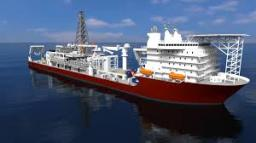We would be pleased if you find an error in the word problem, spelling mistakes, or inaccuracies and send it to us. Thank you!Tips to related online calculators
Check out our ratio calculator.
Tip: Our volume units converter will help you with the conversion of volume units.

## Next similar math problems:

• Geometric progression 2There is geometric sequence with a1=5.7 and quotient q=-2.5. Calculate a17.
• What is 9What is the 15th term; x1=1.5, d=4.5?
• Six termsFind the first six terms of the sequence a1 = -3, an = 2 * an-1
• Calculation of CNCalculate: ?
• AS sequenceIn an arithmetic sequence is given the difference d = -3 and a71 = 455. a) Determine the value of a62 b) Determine the sum of 71 members.
• SequenceFind the common ratio of the sequence -3, -1.5, -0.75, -0.375, -0.1875. Ratio write as decimal number rounded to tenth.
• Researchers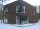Researchers ask 200 families whether or not they were the homeowner and how many cars they had. Their response was homeowner: 14 no car or one car, two or more cars 86, not homeowner: 38 no car or one car, two or more cars 62. What percent of the families
• Converting units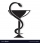Health care professionals frequently convert from one system of measurement to another. Whether you are converting a metric measurement into the household system for a patient or converting within the metric system to enter data in a specific format, the
• Bent scaleMonica weighed 52 kg. Sara 54 kg. Together they weighed 111 kg. They noticed that the weight on the scale was bent. How much did they really weigh?
• Gasoline canisters35 liters of gasoline is to be divided into 4 canisters so that in the third canister will have 5 liters less than in the first canister, the fourth canister 10 liters more than the third canister and the second canister half of that in the first canister
• Warehouses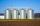In the three warehouses, a total of 70 tons of grain was stored. In the second warehouse was stored 8.5t less and in the third 3.5t more than in the first. How many tons of grain was stored in each warehouse?
• The pigletThe weight of the piglet grew regularly by five kilograms for four months. Determine the proportion by weight of the piglet in each month, if the first weighed 35 kg.
• Vegetable meal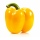The cook was doing meal - in ratio 4: 3: 1 mix tomatoes: pepper: onion. Onions were 5 kg less than peppers. How many kgs of tomatoes did he need to prepare the meal?
• Potatoes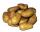Potatoes contain 78.6% starch. How many potatoes need to obtain 27 kg of starch?
• Cross-sections of a coneCone with base radius 16 cm and height 11 cm divide by parallel planes to base into three bodies. The planes divide the height of the cone into three equal parts. Determine the volume ratio of the maximum and minimum of the resulting body.
• Numbers at ratio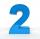The two numbers are in a ratio 3:2. If we each increase by 5 would be at a ratio of 4:3. What is the sum of original numbers?
• Brass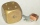Brass is an alloy of copper and zinc in a ratio of 3: 2. How many grams does a component that required 270 g of copper weigh?